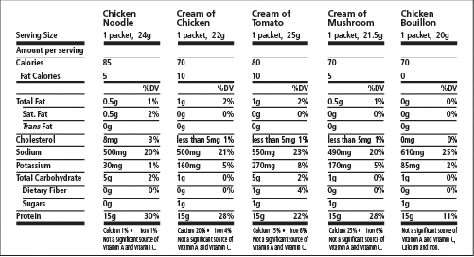# Measurement Mania - Metric Relationships

42 teachers like this lesson
Print Lesson

## Objective

SWBAT explore the relationship between centimeters, decimeters, and meters.

#### Big Idea

In this lesson, students work with partners to determine the number of centimeters in one decimeter and one meter while discovering how to name these relationships as fractions and decimals.

## Warm Up

7 minutes

For this warm up I review the tools we will use today for the lesson, base ten blocks. Students have recently used these tools to help them discover decimal and fraction relationships, so it's important that I spend time today establishing the new use for these tools.

The ones unit will be used as a centimeter, because it's measurements are equal to one centimeter.  The ten's stick will be used as a decimeter because it's length is ten centimeters. (note, I do not tell students that it is 10 centimeters because this is something they will discover in the lesson.) I also introduce students to a meter stick.

In the past many of my students were much more familiar with yard sticks, even commenting that they had a yard stick at their house.  I do hold up a meter stick and a yard stick to make students understand that these two measurements ARE NOT equal. I have found past students to believe that meters and yards are equivalent because students recognize they are both large sticks.

Once most students seem comfortable with the new tools, I move into the concept development portion of this lesson.

## Concept Development

40 minutes

Note: It is expected that students will have prior knowledge/experience related to measuring an object with respect to a particular attribute (for example, length, area, capacity, elapsed time, etc.).

I strongly believe that in order for my students to have a better understanding of the relationships between units, they need to use measuring devices in class lessons. The number of units needs to relate to the size of the unit. They need to discover that there are 12 inches in 1 foot and 3 feet in 1 yard, 10 centimeters in a decimeter, or 100 centimeters in a meter.  When I allow students to use rulers and yardsticks and other tools, they discover the relationships among these units of measurements.

To begin this lesson, I make sure each table has about 100 centimeter cubes, 30 decimeters (ten sticks) and 2 meter sticks.  Students will work with their learning partner in the activity.

Next, I display the metric relationship task activity. metric-relationships activity

I read through the items with students, pointing out that the directions say "explore."  I make sure students understand that the activity is not telling them specifically what to do, but they should explore the materials in order to answer the questions at the end of the activity.

You can see in the two photos below,  students exploring how many decimeters are in one meter. Notice how this assignment lends itself nicely to differentiation.  The students on the left needed to build the 10 decimeter sticks in order to be sure the meter was really 10 decimeters.  The students on the right do not need to build the entire meter stick and were confident in their calculations, noting that there are 10 decimeters in a meter.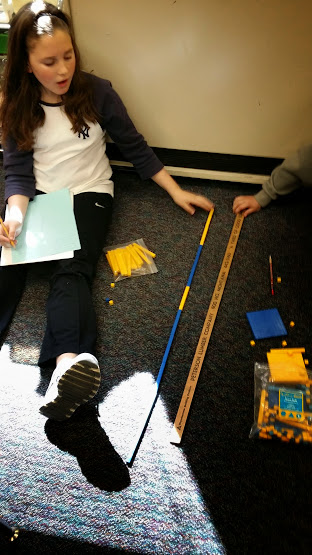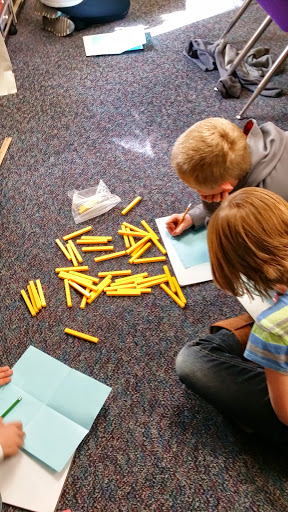Students record the answers to the four questions as they work on a green piece of paper. The last question really gets them to think about the relationship between fractions, decimals, and measurement.

For more information about measurement in fourth grade and common core standards, you can click here to see the measurement progressions document.  ON page 20 you can see a sample table or t-chart - measurment progression document

## Wrap Up - Student debrief

12 minutes

The wrap up of this lesson is so important. While I only planned about 10 to 15 minutes for the wrap up, it is one of the most important parts of this lesson for me.  It is during this wrap up that I MUST help students make connections to prior learning about fractions and decimals.

I start by leading a discussion about what students discovered.  Students respond with telling the class about how many centimeters are in one meter, the number of centimeters in  a decimeter, and the number of decimeters in one meter.  This is still somewhat challenging for them as they try to be accurate in their language and naming the measurement units.

You can see in the photo below a sample of a student work.  This sample is what most students' papers looked like.  Notice in the last box on the right, this students has listed the correct fraction for the relationships.  This was something that I did need to guide and present questions to students in order for them to make sense of what I was asking them to do in this box.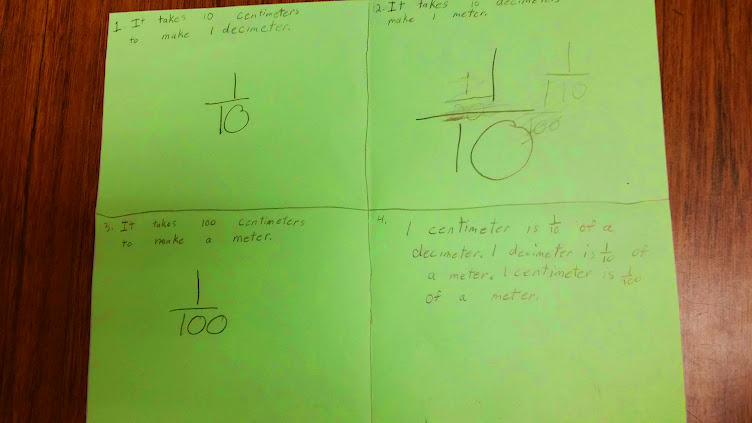During the class discussion, I also make a chart like this with students.  Students record this chart on the back of their green paper.  I talk about with students the meaning of the prefixes of the words kilometer, decimeter, centimeter, and millimeter.  I tell students that "meter" is the base unit and that helps us when we want to know what a word like kilometer is.  When we understand that meter is the base unit, the prefix kilo helps determine how many of the base unit we have. Students begin to understand this concept that kilometer means 1000 meters because of the base unit and the meaning of kilo.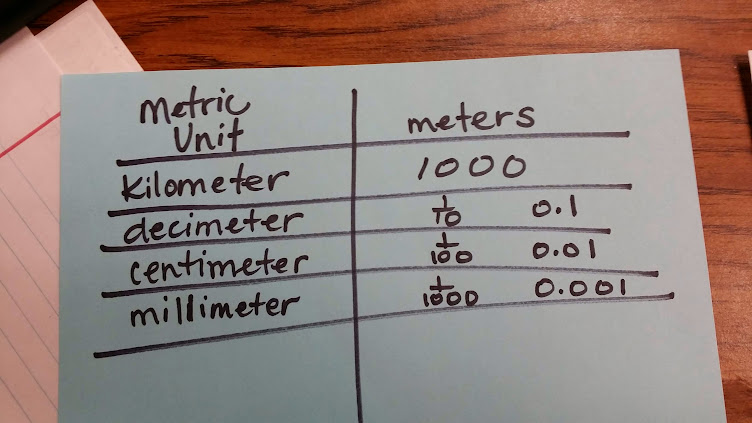I end the wrap up by reminding students that while it can be really fun to measure things and discover how long something is, or how many millimeters are in 20 centimeters, in the "real world" measurement is a tool that helps us be able to do something. I remind students that knowing how to convert units within the metric system is important and that the metric system is used by 95% of the world's population.  All scientists, even those in the United States, use the metric system. Although the metric system is not the official measuring system of the United States, the United States does use it. Most items in the United States are measured and labeled using both customary and metric units.

Students are amazed when I tell them that 95% of the world's population uses the metric system, I then show them this picture and remind them that the United States does too.  I remind them that grams (g) are in the metric system.  Students leave this lesson usually very excited to go home and check all their food labels to see if I'm right.  I predict I'll have some students in my room bright and early telling me stories of digging through pantries.